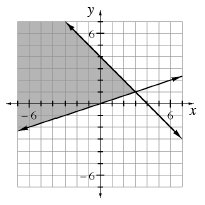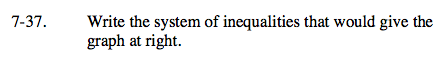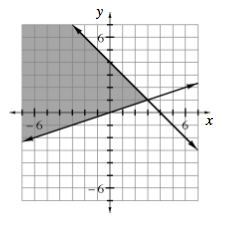Home > A2C > Chapter 7 > Lesson 7.1.3 > Problem7-37

7-37.

Write the system of inequalities that would give the graph below. Homework Help ✎Find the y-intercepts and slopes of the lines by looking at the graph.

Determine the equations of the lines and then write the inequalities.

y ≤ −x + 4